# How to convert text to number in Excel

The tutorial shows many different ways to turn a string into a number in Excel: Convert to Number error checking option, formulas, mathematic operations, Paste Special, and more.

Sometimes values in your Excel worksheets look like numbers, but they don't add up, don't multiply and produce errors in formulas. A common reason for this is numbers formatted as text. In many cases Microsoft Excel is smart enough to convert numerical strings imported from other programs to numbers automatically. But sometimes numbers are left formatted as text causing multiple issues in your spreadsheets. This tutorial will teach you how to convert strings to "true" numbers.

## How to identify numbers formatted as text in Excel

Excel has an inbuilt error checking feature that alerts you about possible problems with cell values. This appears as a small green triangle in the top left corner of a cell. Selecting a cell with an error indicator displays a caution sign with the yellow exclamation point (please see the screenshot below). Put the mouse pointer over the sign, and Excel will inform you about the potential issue: The number in this cell is formatted as text or preceded by an apostrophe.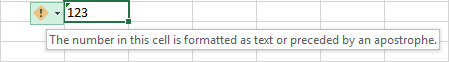In some cases, an error indicator does not show up for numbers formatted as text. But there are other visual indicators of text-numbers:

 Numbers Strings (text values) Right-aligned by default. If several cells are selected, the Status Bar shows Average, Count and SUM. Left-aligned by default. If several cells are selected, the Status Bar only shows Count. The Number Format box displays the Text format (in many cases, but not always). There may be a leading apostrophe visible in the formula bar.

In the image below, you can see the text representations of numbers on the right and actual numbers on the left: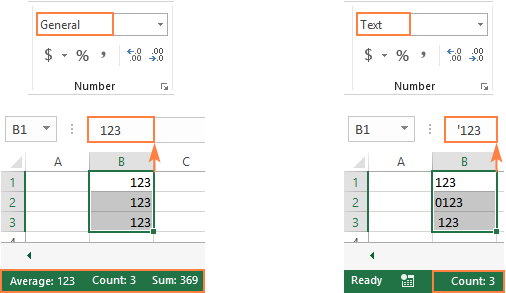## How to convert text to number in Excel

There are a handful of different ways to change text to number of Excel. Below we will cover them all beginning with the fastest and easiest ones. If the easy techniques don't work for you, please don't get disheartened. There is no challenge that cannot be overcome. You will just have to try other ways.

### Convert to number in Excel with error checking

If your cells display an error indicator (green triangle in the top left corner), converting text strings to numbers is a two-click thing:

1. Select all the cells containing numbers formatted as text.
2. Click the warning sign and select Convert to Number.

Done!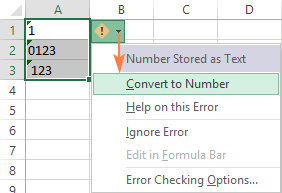### Convert text into number by changing the cell format

Another quick way to convert numerical values formatted as text to numbers is this:

1. Select the cells with text-formatted numbers.
2. On the Home tab, in the Number group, choose General or Number from the Number Format drop-down list.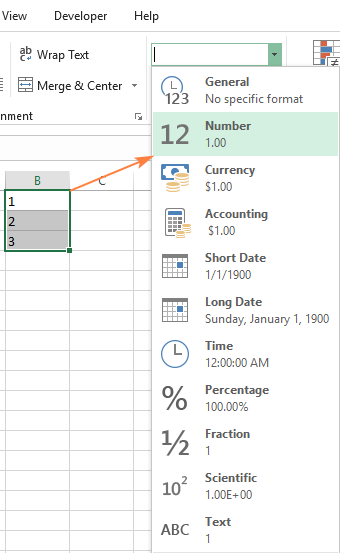Note. This method does not work in some scenarios. For example, if you apply the Text format to a cell, enter a number, and then change the cell format to Number, the cell will remain formatted as text.

### Change text to number with Paste Special

Compared to the previous techniques, this method of converting text to number requires a few more steps, but works almost 100% of time.

To fix numbers formatted as text with Paste Special, here's what you do:

1. Select the text-number cells and set their format to General as explained above.
2. Copy a blank cell. For this, either select a cell and press Ctrl + C or right-click and choose Copy from the context menu.
3. Select the cells you want to convert to numbers, right-click, and then click Paste Special. Alternatively, press the Ctrl + Alt + V shortcut.
4. In the Paste Special dialog box, select Values in the Paste section and Add in the Operation section.
5. Click OK.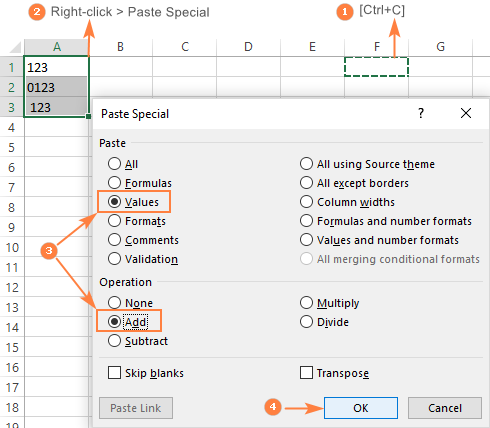If done correctly, your values will change the default alignment from left to right, meaning Excel now perceives them as numbers.

### Convert string to number with Text to Columns

It is another formula-free way to convert text to number in Excel. When used for other purposes, for example to split cells, the Text to Columns wizard is a multi-step process. To perform the text to number conversion, you click the Finish button in the very first step :)

1. Select the cells you'd like to convert to numbers, and make sure their format is set to General.
2. Switch to the Data tab, Data Tools group, and click the Text to Columns button.
3. In step 1 of the Convert Text to Columns Wizard, select Delimited under Original data type, and click Finish.

That's all there is to it!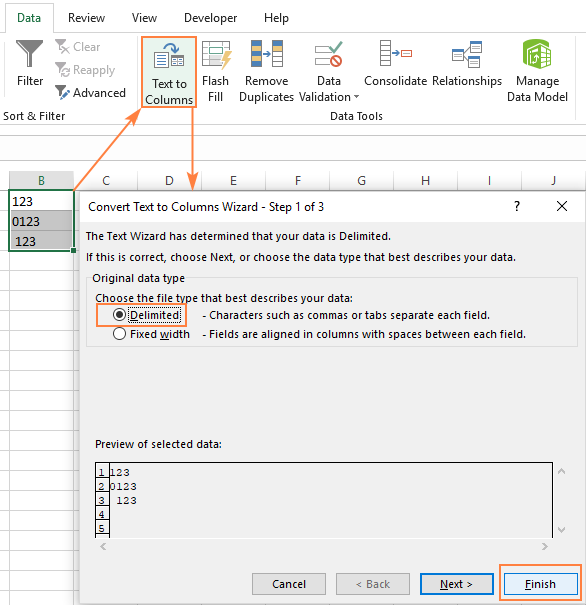### Convert text to number with a formula

So far, we have discussed the built-in features that can be used to change text to number in Excel. In many situations, a conversion can be done even faster by using a formula.

#### Formula 1. Convert string to number in Excel

Microsoft Excel has a special function to convert a string to number - the VALUE function. The function accepts both a text string enclosed in quotation marks and a reference to a cell containing the text to be converted.

The VALUE function can even recognize a number surrounded by some "extra" characters - it's what none of the previous methods can do.

For example, a VALUE formula recognizes a number typed with a currency symbol and a thousand separator:

`=VALUE("\$1,000")`

`=VALUE(A2)`

To convert a column of text values, you enter the formula in the first cell, and drag the fill handle to copy the formula down the column: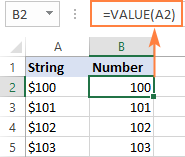#### Formula 2. Convert string to date

Apart from text-numbers, the VALUE function can also convert dates represented by text strings.

For example:

`=VALUE("1-Jan-2018")`

Or

`=VALUE(A2)`

Where A2 contains a text-date.

By default, a VALUE formula returns a serial number representing the date in the internal Excel system. For the result to appear as an actual date, you just have to apply the Date format to the formula cell.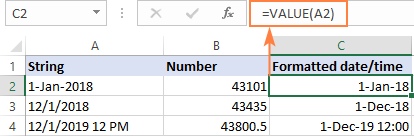The same result can be achieved by using the DATEVALUE function:

`=DATEVALUE(A2)`

#### Formula 3. Extract number from string

The VALUE function also comes in handy when you extract a number from a text string by using one of the Text functions such as LEFT, RIGHT and MID.

For example, to get the last 3 characters from a text string in A2 and return the result as a number, use this formula:

`=VALUE(RIGHT(A2,3))`

The screenshot below shows our convert text to number formula in action: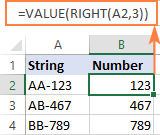If you don't wrap the RIGHT function into VALUE, the result will be returned as text, more precisely a numeric string, which makes any calculations with the extracted values impossible.

### Change Excel string to number with mathematic operations

One more easy way to convert a text value to number in Excel is to perform a simple arithmetic operation that does not actually change the original value. What can that be? For example, adding a zero, multiplying or dividing by 1.

`=A2+0`

`=A2*1`

`=A2/1`

If the original values are formatted as text, Excel may automatically apply the Text format to the results too. You may notice that by the left-aligned numbers in the formula cells. To fix this, be sure to set the General format for the formula cells.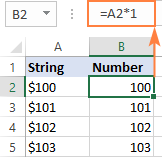Tip. If you'd like to have the results as values, not formulas, use the Paste Special feature to replace formulas with their values.

That's how you convert text to number in Excel with formulas and built-in features. I thank you for reading and hope to see you on our blog next week!

## You may also be interested in

1. I have already tried something like 10 methods to convert cells like this
1 959,94
(where the space is the thousand separator and the comma is the decimal separator) to numbers, but nothing has worked. What would work?

• Hello!
Replace the space with nothing using the SUBSTITUTE function. Then use any mathematical operation or other method described in the article above to convert text to a number.

=--SUBSTITUTE(A1," ","")

Read more How to remove special (unwanted) characters from string in Excel.

• This is odd: my fresh Excel (Win 11) does not accept colons to separate arguments of the function but requires me to use semicolons. I can do that but when I wrote
=SUBSTITUTE(A1," ","")
it gave me still 1 959,94.

Only after I wrote
=SUBSTITUTE("1 959,94"," ","")
it gives me 1959,94
but there is no sense to write the contents of all the cells I have to convert to numbers by hand.

What to do?

• I cannot know what delimiters you use in formulas and what decimal separator is set in your regional Windows settings. Replace comma delimiters in the formula with semicolons, if necessary.
If you use a dot as a decimal separator, replace the comma with a dot.

=--SUBSTITUTE(SUBSTITUTE(A1," ",""),",",".")

• My system separators that my Excel uses are the comma for the decimal separator and the space for the thousands separator.

I have still the problem that when having
1 959,94 in A1 and writing
=SUBSTITUTE(A1," ","")
the function gives me 1 959,94.

Only after writing
=SUBSTITUTE("1 959,94"," ","")
the function gives me 1959,94.

The numbers in my original data (a table copied from the internet using copy-paste) that are below 1000 (the numbers without the thousand separator) behave nicely as numbers without any tricks. _All_ the copy-pasted numbers align right in their cells. Only the numbers that include the thousand separator can't be used in calculations as their cause #VALUE! error. What could be the cause for this?

• As mentioned in the article above, perform a mathematical operation on the text to convert it to a number. Read the article above carefully. Аlso take a look at my answers.
For example,

=--SUBSTITUTE(A1," ","")

• As I have tried to explain when having
1 959,94 in A1 and writing
=SUBSTITUTE(A1," ","")
the function gives me 1 959,94, i.e., the function does not remove the space. And if I try to for example multiply the result by 1, the result is the error
#VALUE!

I have managed to remove the space only if I type the first argument of the function by hand like this:
=SUBSTITUTE("1 959,94"," ","")

• Try this
=VALUE(CONCAT(TEXTSPLIT(CONCAT(TEXTSPLIT(A1," ")), ",")))

• It works
=VALUE(CONCAT(TEXTSPLIT(CONCAT(TEXTSPLIT(A1," ")), ",")))/100

2. I got it just by typing
=[cell with number in text format]+0

• After exhausting all the methods I could find on the web, I solved the problem by copying and pasting the problematic data to MS Word, then re-exporting to Excel as Text. It was immediately recognizable by Excel as values for purposes of applying formulas, summation, etc.

• this works for me. thanks for your input

3. this is not working in any of the solutions/function provided above . the excel i have uses "," for thousand separator and "." for decimals
2.866.794,97
321.279,70
321.279,70
45.647,28
45.647,28

4. some times you need change seprator to"."
may be before the change to text ,that seprator was "." but now seprator is"/"
there is errore for change to value

5. How can I convert a number (I get it from data from WEB) to Pivot table? Number in data is not appearing in pivot table. I used convert text to columns wizard and it work but every time I refresh the data, I need to go to convert text to columns wizard again.

6. I want to convert from this value 1-120-00001297-7 to 1120000012977. How can i do? Thank you.

7. Great explanation, thank you

I encounter this problem with different way, I downlaoded a sheet that includes column "Budget", the cells in this colum are formatted as currency and it is left aligned, and the status bar shows only count. I tried to convert it to numbe by using Value function but it gives me error, the only way that worked with me, is selecting the cells and replace \$ to blank, this makes the figures inside the cells numbers.
Is there any explanation for this?

• Hello!
The \$ sign in the cell was not obtained using number formatting. It is written as text. So when you remove it, the value becomes a number.

8. This has been such a mightmare. I finally got the following to work. Following all your suggestions and more, I just replace-all'd elimination of "\$" and "." and ".00" to get 2, 3, 4, and 5 digit numbers that stubbornly refused to go to numeric. I extracted from the left the correct number of digits -- eg, 50 wants =let(cell,2) -- then I had to =trim(new cell) **even though there is no apparent space anywhere in sight**. Still it's not numeric but at that point you can multiply by 1 and turn it into a freaking number: yay!!!

You cannot imagine how long it has taken to get this to work.

And you have to manually reset the number of digits to extract. extracting more than the visible digits and then triming, just doesn't work. (@@) go figure.

• Did you manage to resolve this? I am having the same issue here.

• Hi!
Explain your problem in more detail and give an example of the data and the desired result.

9. Hello, I'm trying to erase a space before a number (for thousands of cells which I got from another file) but I can't do it:
Example: a cell contains a space before a number 1, and I need it to be just number 1, without a space before it.
The problem with the number 1 after a space is that excel consider it a a text, the SUM of it is 0.
When I overwrite a 1 in that cell which contains a space before the number 1, it converts to a number and SUM operates normally; but I can't do it cell by cell for thousands of cells.

• Hello!
Have you tried the ways described in this blog post? Try Paste Special or Convert to Number as described in this article. These operations can be performed with the entire range of cells.

I tried Paste Special, but when I copied the cell it copied only those which were numbers already, and didn't copy the other "numbers" which doesn't work as numbers.

When I look at the numbers in the columns I notice that the same number is a little bit (one space) to the left of the "so called number" (which cannot be added, etc)
If I look at that cell in particular, which contains for instance 145 it has a space before the number,
but if I replace that writing the number 145 over it, will not have any space before it and then it will work fine as a number.

Can't understand what kind of format those so called numbers have.

• Hi!
If I understood correctly, you are copying the cells with your values, in which the numbers are written as text. Read the instructions carefully. Copy only one empty cell and then use Paste Special.

• Do a 'find and replace' and put a space character in the find field and nothing in the replace field. It will apply to everything though....

• Hello Tommy,
For example, if the column containing the numbers is A, and the first cell with data is A2 [not counting the header row], you should:
1. Create (Insert a new column (B in our example)
2 . In column B2, type: TRIM(A2)
[this will give the output of the content of the content of cell A2 without any leading or trailing spaces]
3. You can then fill the formula down by double-clicking the fill handle or any other way.
[this will make the entire column B the same as A without any leading spaces]
4. You can then copy column B and paste it in A as VALUES using the PASTE SPECIAL.

I hope this helps.

10. Hello,
I am trying to convert Satisfaction surveys from text to numbers, to avoid manual converting for receiving a high number of surveys in a weekly. I was provided with the following formula with little to no information on how to add it on the exported repot. There is a main sheet, but the data needs to be add once the exported sheet is completed. The formula provided is below and I am trying to convert only one column from text to numbers.
=IF(T3489="Extremely dissatisfied",1,IF(T3489="Somewhat dissatisfied",2,IF(T3489="Neither satisfied nor dissatisfied",3,IF(T3489="Somewhat satisfied",4,IF(T3489="Extremely satisfied",5,0)))))

Hoping to hear back!

• Hi!
I don't quite understand how I can help. I can't see your data. Add this formula to your worksheet. Change the cell address if necessary.

• I think the above formula is just working fine & is able yo convert the col containing the survey response to numbers. What is the exact issue, unable to comprehend from your message above.

11. 14811.10
10363.44
1 059.00
-4 534.00
-10 104.90

How to get this into numbers ? Text to Column doesnt work

• Hello!

=--SUBSTITUTE(A1," ","")

If you use comma as decimal separator then use this formula

=--SUBSTITUTE(SUBSTITUTE(A1,".",",")," ","")

I'd recommend you to have a look at our Convert Text tool that can help you convert numbers stored as text to numbers. It is available as a part of our Ultimate Suite for Excel that you can install in a trial mode and check how it works for free.

12. I have a list of names in excel which im looking to formulate into a list of numbers
All names are differnt but im looking them to change into number formatt 1-8

• Hi!
Unfortunately, without seeing your data it is difficult to give you any advice. Please provide me with an example of the source data and the expected result.

13. How do I convert a duration to show a number:
example:
0:07
0:15
0:35
And convert these to read as:
0,07
0,15
0,35

• Hi!
Please check the formula below, it should work for you:

=SUBSTITUTE(TEXT(A1,"h:mm"),":",",")

14. I have non numerical data (responded in monkey survey) the responses are in word i.e. agree, strongly agree, disagree, strongly disagree for many questions. I have exported the data of the survey to Excel sheet and all the responses are in word (string). I need to analyses basic statics corelating it with one variable (like male/female respondents). How do I convert this strings to numeric for analysis in excel?.

• @Alexander Trifuntov - Thank you very much, this has been very helpful and work simplified. Thank you very much once again.

• Thank you Alexander, Very helpful

15. Hello
I have
1819VJO2045643_1
2021RDO456326_1
1920TDO785432_2
Etc
Etc

Like this data with me i want to convert this string in to number or unique numbers how to convert please help me
Thanks

• Hello!
To extract all numbers from text, try this formula:

=CONCAT(IF(ISNUMBER(--MID(A1,ROW(\$1:\$94),1)),MID(A1,ROW(\$1:\$94),1),""))

If this is not what you wanted, please describe the problem in more detail.

• Sir
how to find numbers and text

Expl

sunil12542kumar

I WANT RESULT

Sunil KUMAR 12542

• Hello!
The following tutorial should help to extract numbers: Extract number from string in Excel.
If you extract the numbers into a separate cell (for example, B1), then using the SUBSTITUTE function you can remove them from the text:

=SUBSTITUTE(A1,B1," ")

You will get text "sunil kumar".
Merge text and numbers using the CONCATENATE function or the & operator.

16. I have cells containing a simple subtraction like 12-9. It needs to display like that so I have applied Text formatting to it. The cell next to it needs to display the calculation - in this case 3. Excel converts it to a date and displays 44451. The 12-9 needs to be changeable and not require reformatting after data entry. Hope you can help - thanks

17. Hi,
I am trying to convert a value with dollar sign in text to Numeric but Value Function is giving error instead of giving 400 & 500 as results. Excel doc also says this should work. What may be the probable reasons.
I am posting the exact code below

A B C
1. Name Money Won Value
2.
3. Mico \$400 #VALUE! #=VALUE(B3)
4. Noah \$500 #VALUE! #=VALUE(B4)

• Hi!
What are you trying to convert to a number, Mico \$400 or \$400? What formula are you using in cell B3? If you are trying to convert the value "\$400" to a number, check which currency is set by default in the regional settings of Windows.

18. Dear all, thank you for all these tips but it is not working for me. I need to change numbers stored as text into general format in order to be able to use a VLOOKUP formula. When I change my text into general I notice that the value change as well. For instance, it changes 92753660758293 into 92753660758290... How can I keep the right value? Many thanks for your answer.

• Hello!
I don't know how you turn text into numbers. There are many ways. I have not been able to replicate your problem. However, keep in mind that the maximum number of numeric digits in Excel is 15.

19. A1= 1
A2 = (1+5)/2 as text
A3 = (2+4)/2 as text
in A4 i need the formulated A1*A2*A3
A2 & A3 only can be write as text type

• Hi!
You can only convert the text in a cell to a formula using a VBA macro.

• I have same issue

20. I have a large file with a column of data where I want to convert a 5 digit/character code alphanumeric text to number. Your instruction handles most of these cells in my file, but not for instances with an embedded alpha "E" character in the string. This column has 5-character codes that I wish to convert to number for a vlookup application. An example: a code of 71E62. I cannot get that cell (and many others) to convert to a number.

• Hi!
I cannot guess which number the text "71E62" corresponds to.

21. I am still battling with this:
There are a number of tricks you can use if you have a column of numbers in a format that Excel does not recognise as numbers. For example, let's say you have a column of numbers where some idiot has typed each number in using a comma as the thousands separator like this:

52,321.45
17,615.28
19,785.98
41,963.49

If your version of Excel does not use commas for the thousands separator, (mine is et to use the apostrophe - ' -), or if some dummy actually typed in the commas, you can still quickly convert the entire column of text-numbers to numbers by using the Data - Text to columns function:

Highlight the entire column.
Click text to numbers
Select "Delimited" and hit next.
Uncheck all the delimiters and hit next
In the advanced box it asks for the decimals separator and for the thousands separator.
Enter the separator you see on the screen (NOT the separator that you want). So in the above example you would put a comma as the thousands separator, and a full-stop as the decimal separator.
click OK and finish.

It works !!! - until the "text number" is in 1000's
ie: R35,06 text converts to R35.06 number and
R128,71 text converts to R128.71 number

BUT -
R4 020,40 text says as is and does not convert

• Hello!
You can remove spaces from text using the formula

=SUBSTITUTE(SUBSTITUTE(B1," ",""),",",".")

22. 238453758
182121050
180284560
190244150
219852959
68828553
42000000

how to Change above string to numbers? thanks

23. Hello All,

I have data with 10 digit followed by X. I need a digit in place of X.

Example: 0123456789X is the data and i need the output to be like 01234567890, 01234567891,.......0123456789

• Hello!
If I understand your task correctly, the following formula should work for you:

="0123456789"&SEQUENCE(100,1,0,1)

24. how do I convert all text strings to numbers in a column in Excel sheet
'Php200.00
'Php500.00
'Php200.00
'Php100.00
'Php3,432.00
Thank you.

• Hello!
If I understand your task correctly, the following formula should work for you:

=--MID(SUBSTITUTE(A1,",",""),4,20)

Hope this is what you need.

25. Thanks.

26. Thanks a lot

27. none of these worked for me. I have just numbers copied from powerpoint into excel. I've used, trim, value, left, text to columns, and just simply changing it, and none of these are working.

• Hello!
You can save the Powerpoint file as PDF and then import it into Excel as written in this article - Converting PDF files to Excel

28. Grande! Thanks!

29. 39.2 -90.1 I have two values but i keep to both values in two different column how to do?

• Hi,
Please check out this article to learn how to split text string by delimiter. Then you can convert two text strings to a numbers as described in this tutorial above.

30. Hi!
I work on spreadsheets daily, but all this needed info is tiresome!!
I need a way where I can type minimum and it says it all...
Like for instance: a column has different things like; interested, not interested, phone tomorrow, phone June, etc.
Lazy me wants to connect each to a number and by pressing that number, it will say "Interested" or how ever it may be...
I also need to know how to get the one column to add automatically say three days for follow-up call or to highlight the June call, that needs to be made, appear more urgent as we approach June, for instance...

Any possible way to assist, please?

Thank you kindly
Annatjie

31. How can I convert these numbers? I tried every suggestion above, but it did not work

0.9587628865979382

32. Hi. How do I get a column of written data selections (unlimited rows but 10 or 12 choices, eg different pathology types, to be converted to nunbers. Say, infection = 1, trauma = 2 etc.....

33. I have a column of numbers that have an "AUD" reference at the end of each number. As a result it is not recognising them as numbers and I cannot tally the column. How do I remove the "AUD" reference from each cell to enable it to be added as numbers?

• Hello!
If I understand your task correctly, you can use SUBSTITUTE function to remove unnecessary text and extract number:

=--SUBSTITUTE(A10,"AUD","")

If you have other questions, don't hesitate to ask.

34. Thank you so much

35. thank you

36. Great Article ....

37. in excel if cell gets value of numer as text than
Befor enting the value in cell use =ABS(cell number)

38. use below view for more clarity
.................................... Total
1 20 0 10 20 20
2 25 25 20 10 25
3 15 25 8 40
4 40 200 10 30 40
5 10 100 20 10 110
6 30 100 6 20 30
7 5 100 10 10 5
8 0 100 8 20 0
9 20 100 8 20
10 25 200 0 30 25

39. I have to get total of each row in below. I used "auto sum " to get the result, but it gets wrong value for each raw. How can i fix this, pls. help
Total
1 20 0 10 20 20
2 25 25 20 10 25
3 15 25 8 40
4 40 200 10 30 40
5 10 100 20 10 110
6 30 100 6 20 30
7 5 100 10 10 5
8 0 100 8 20 0
9 20 100 8 20
10 25 200 0 30 25

• Hello!
What formula are you using? Formula =SUM(A1:E5) doesn't work? Explain in detail what result you want to get.

40. How to convert 89000478513C,890004687210 to 8900100047851303,8900100046871010 respectively and so on

• Hello!
To replace a letter with its number in the alphabet, use the formula

=CONCAT(IFERROR(IF(ISNUMBER(--MID(A1,ROW(\$1:\$93),1)), MID(A1,ROW(\$1:\$93),1), IF(CODE(MID(A1,ROW(\$1:\$93),1))-64<=0, MID(A1,ROW(\$1:\$93),1), CODE(MID(A1,ROW(\$1:\$93),1))-64)),""),"")

This is the formula for uppercase letters. If you need to replace lowercase letters, then instead of 64 use 96 in the formula

41. how to convert area to numbers
exp.area= 79.84 convert into number?

42. Hi,
How do I recode a drop-down list in Excel, in other words assign a code number for each of the drop down responses? I've tried several of the options but they haven't worked.
Thanks

• Hello Ellen!
You can use the Combo Box (menu Developer - Insert - Form Controls - Combo Box). It returns the number of the selected item from the drop-down list to the cell you designated

43. I have
AA 8881/17
BR 078/16
BR 078/18
BR 078/19
CZ 458/18
EK 148/15
EK 148/15
EK 9287/18
KL 0427/15
KL 0427/18
and i need only the last 2 digits or to be sorted from the last 2 digits how can I do it?

• Hello!
To extract the last 2 digits from the text, use the RIGHT function
Text Result -

=RIGHT(A1,2)

Number result

=--RIGHT(A1,2)

44. How we can data in number when some data copied with table and pasted in excel now that numbers are in text and before starting numbers many spaces are there
how we can do convert such cases in number format

45. Hi,
I cannot use vlookup formula when numbers are presented as text.
Example I have:
0456
1247
1234/1,
and I need to have the values as text, so I can sort them A to Z. I always get the error massage, when use vlookup formula.
Any help?

46. I have a column on the left that goes from 0-60. The column on the right contains a formula that reads: =COUNTIF(CY\$4:CY\$66,0
I put \$ so that those inputs don't change but I'd like for the 0 to change to 1, 2 and so on.
So going down the column it should look like this:
=COUNTIF(CY\$4:CY\$66,0
=COUNTIF(CY\$4:CY\$66,1
=COUNTIF(CY\$4:CY\$66,2
=COUNTIF(CY\$4:CY\$66,3
And so on.
That way I don't have to go into each cell to change each number individually. The reason I have to change it is because I have to add and delete rows which causes for there to be a gap which I then have to fix. I'm sure there's a way. Please help!

• I recommend using the ROW function as a counter.

=COUNTIF(CY\$4:CY\$66,0)

is in cell D2 (i.e., line 2) then change the formula

=COUNTIF(CY\$4:CY\$66,ROW()-2)

After that you can copy this formula down along the column.
Hope this is what you need.

47. Hi all.
Need help on formula.
E.g.
In cell A2, there is 4 digit no. "1234"; cell A3, "2345".
In cell B2, there is a text "M" and cell B3 text "C".
How to form a formula in cell C2 and C3 that the output are "MM-181234-01" and "CC-182345-01" respectively.

• Hello Judith!

=CONCATENATE(B2,B2,"-18",A2,"-01")

Hope this is what you need.

48. I have a number 328,345 in General format
Somehow I looked some of the hidden characters it looks like "328,345 " notice the space
I used clean, trim and value in all the nine orders but to no avail can i get that number to allow me to do any number functions like add.
Any ideas

• Hello Fran!
If I understand your task correctly, the following formula should work for you:

=VALUE(SUBSTITUTE(A1,",",""))

I hope this will help, otherwise please do not hesitate to contact me anytime.

49. Please, kindly suggest how to convert all text strings to numbers in a column in Excel sheet? Example:
\$3,51,670
\$4,24,980
\$2,31,650
\$5,29,810
\$3,12,980
\$2,47,360
\$9,23,840
\$1,26,120.
I have tried all the suggestions stated above but it still doesn't recognize it as a number when I want to calculate or find the maximum of some numbers and it is included.

• Hello!
﻿If I understand your task correctly, the following formula should work for you

=--SUBSTITUTE(SUBSTITUTE(C21,",",""),"\$","")

I hope this will help

50. Hello,
I'm working with Geodesy and obviously excel is a part of it since i insert my points in measurement device. So in one of the columns i have list of numbers 145,987 etc. and it appears to be correctly written number, until i select that cell and in the function bar it appears 145987. I've tried changeing to text, customize format cells general, even entered formulas, and in options changed that thousands be marked as , and I manage to change number with a comma 145,987 but in function bar it says 145987.